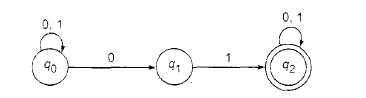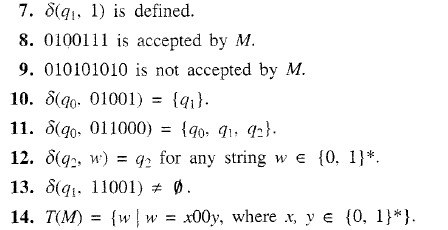##### Which of the following is true for given finite automata?True or false pls explain with valid reason point number 7,9,11,13,14##### 1Comment
shivani
15 Aug 2017 05:48 pm

point 7 δ(q1,1) is defined : true // as  δ(q1,1) =q2

point 9  010101010 is not accepted by M  : false  //δ(q0,010101010) can be written  δ((q0,010101),010) =q1 which is a final state

point 11  δ(q0,011000) ={q0,q1,q2} : true // as  δ((q0,0),11000) =  δ((q1,1),1000) = δ(q2,000) = q2

point 13  δ(q1,11001) ≠ Ø : true // as δ(q1,11001) = q2